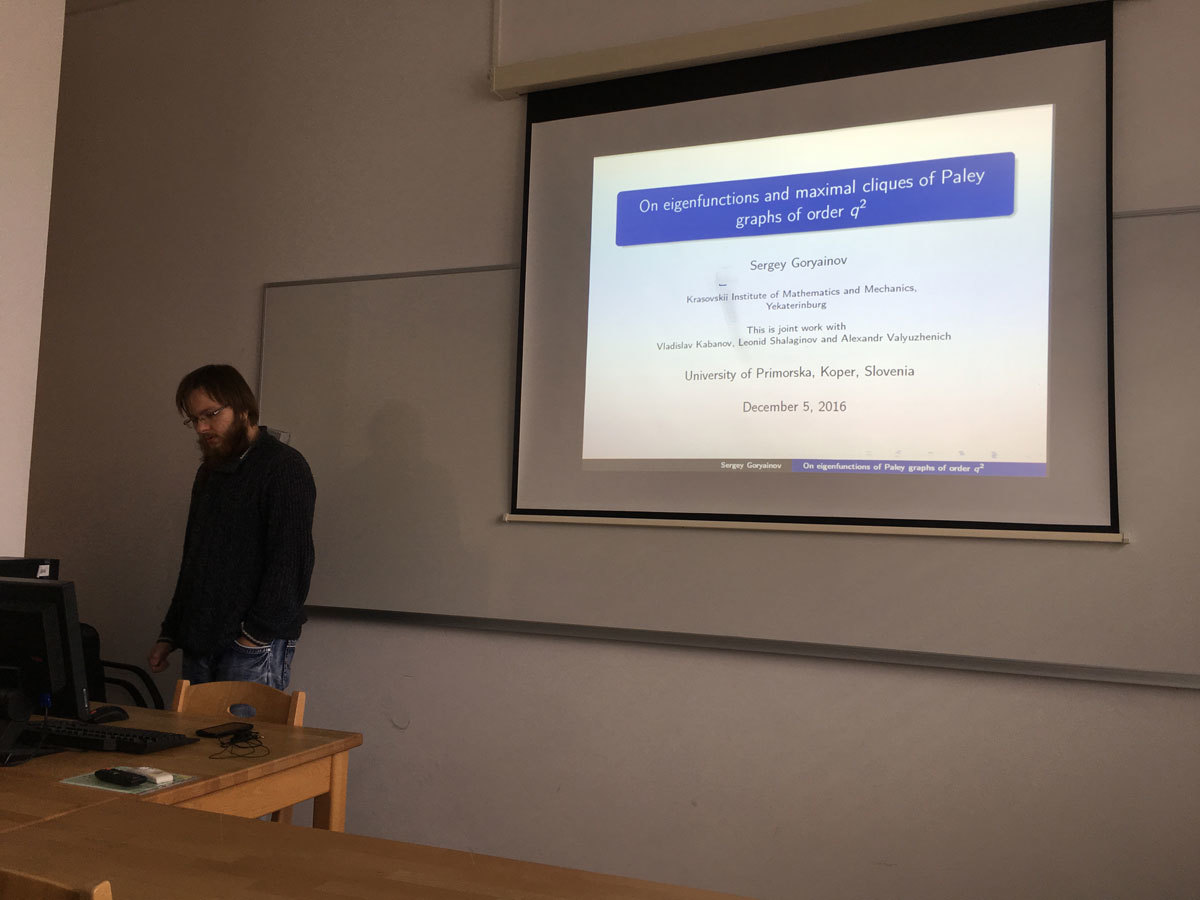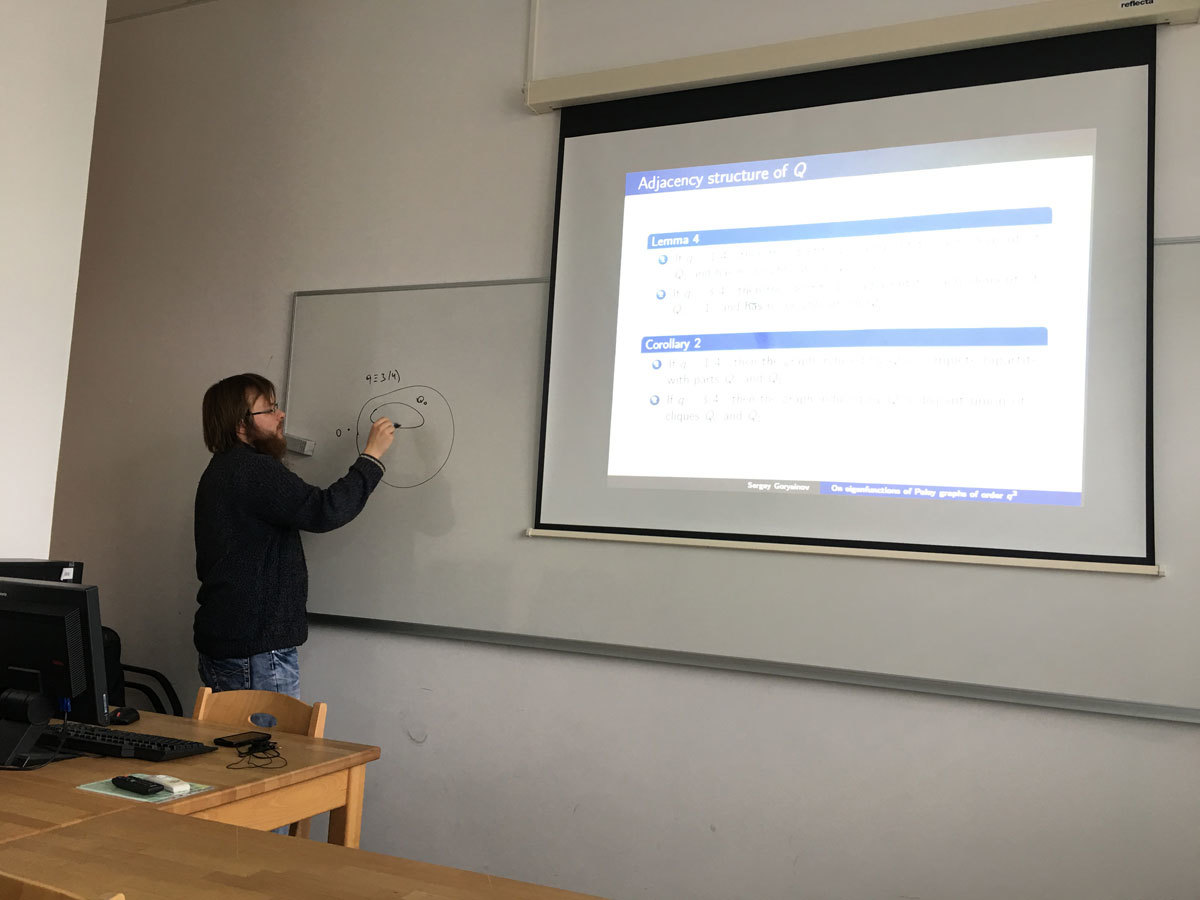Univerza na Primorskem Fakulteta za matematiko, naravoslovje in informacijske tehnologije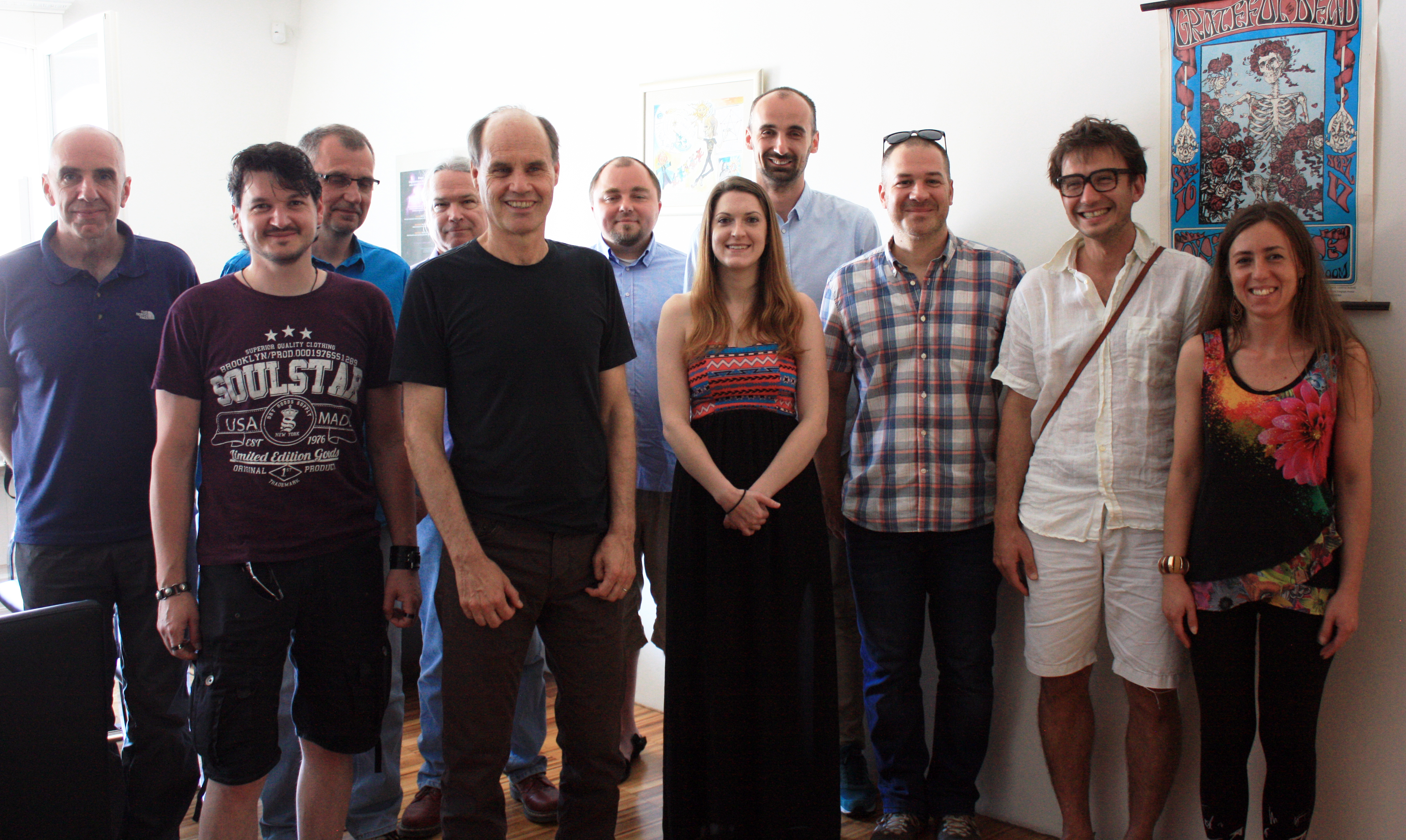SI | EN# Raziskovalni matematični seminar - Arhiv

 2023 2022 2021 2020 2019 2018 2017 2016 2015 2014 2013 2012 2011 2010
 1 2 3 4 5 6 7 8 9 10 11 12
Datum in ura / Date and time: 19.12.16
(10:00-11:00)
Predavalnica / Location: FAMNIT-POŠTA
Predavatelj / Lecturer: Samir Hodžić (UP IAM & UP FAMNIT)
Naslov / Title: Characterisation of Generalised Bent Functions and Some Other Topics Related to Cryptography.
Vsebina / Abstract:

This is a presentation of the PhD thesis topic. The PhD thesis will consist of two parts: Generalized bent (gbent) functions (as a main part) and other topics related to cryptography.

Having the application in OFDM and MC-CDMA modulation techniques, which suffer from relatively high peak-to-mean envelope power ratio (PMEPR), in 2007 K. U. Schmidt proved that PMEPR is minimized if and only if a gbent function is used in OFDM/MC-CDMA systems. In the PhD thesis we will provide a full characterization of gbent functions (mappings from F^n_2--> Z_q), where we show that in algebraic sense they correspond to affine spaces of bent or semi-bent Boolean functions which satisfy certain properties related to Sylvester-Hadamard matrices.  Moreover, we introduce the notion of Z_q-bent functins (as a subclass of gbent functions) which correspond to relative difference sets/ vectorial bent functions. For even number of input variables n, we determine the dual function of a gbent function, and depending on the parity of n we will prove that Gray maps of gbent functions are plateaued Boolean functions.

Apart from related topics, we consider three problems. First, we consider the problem of optimizing the placement of tap positions in LFSR-based stream ciphers. Analyzing the GFSGA attack we provide two algorithms for taps selection. We show that our algorithms may provide a significant improvement of resistance of the LFSR-cipher (which employs a filtering function) to GFSGA-like attacks comparing to relative difference sets. In addition, two new modes of the GFSGA attack are developed.
Then, we provide an algorithm for estimating the resistance of a random Boolean function to (Fast) Algebraic attacks (for relatively large number of input variables, say n>30 -- which appears to be a hard problem).  The complexity of our algorithms is estimated as O(n^2 2^n), which is much less then previous known algorithms.
At the end, we consider the problem of finding polynomials over finite fields which cannot possess  linear structures. Such polynomials provide an optimal resistance to differential cryptanalysis. We identify several infinite classes of such polynomials, and we derive the upper bound on the degree of so-called planar mappings.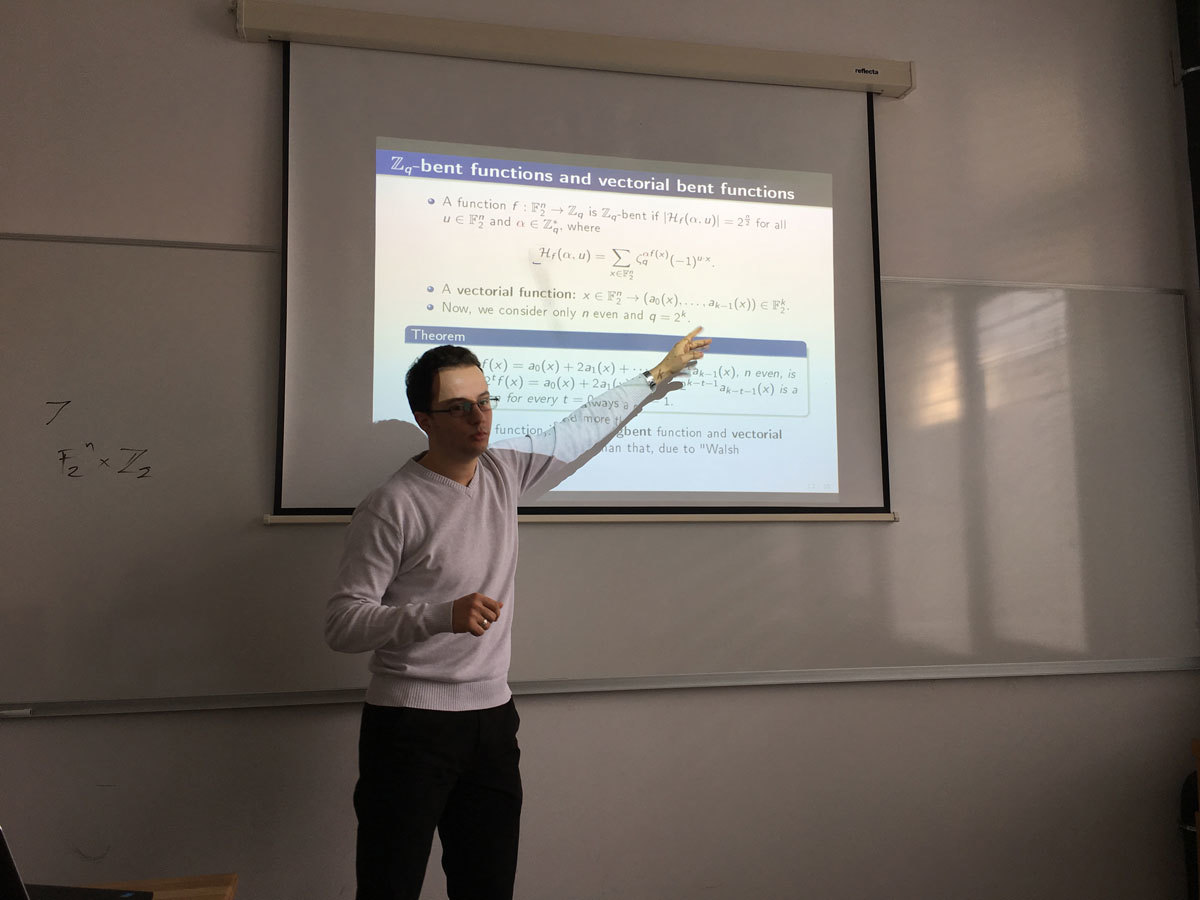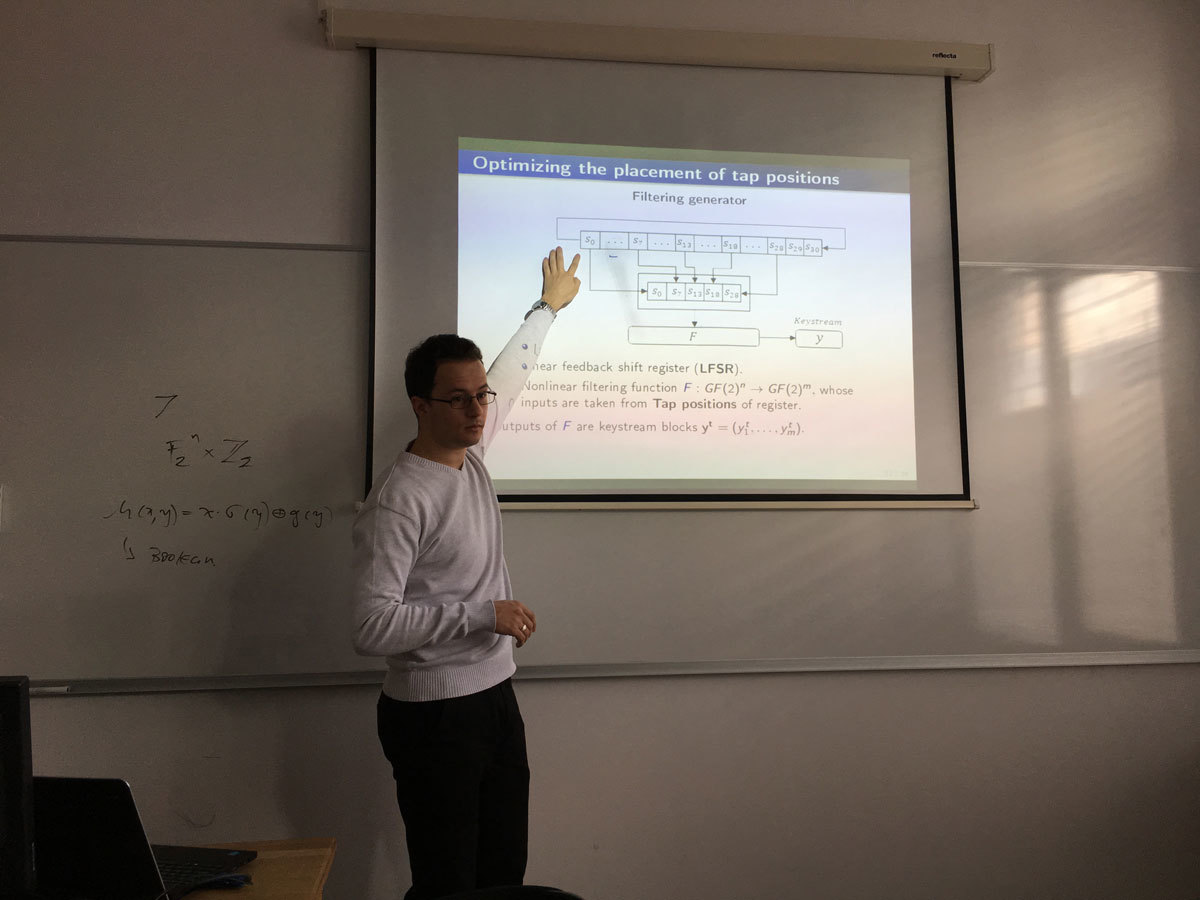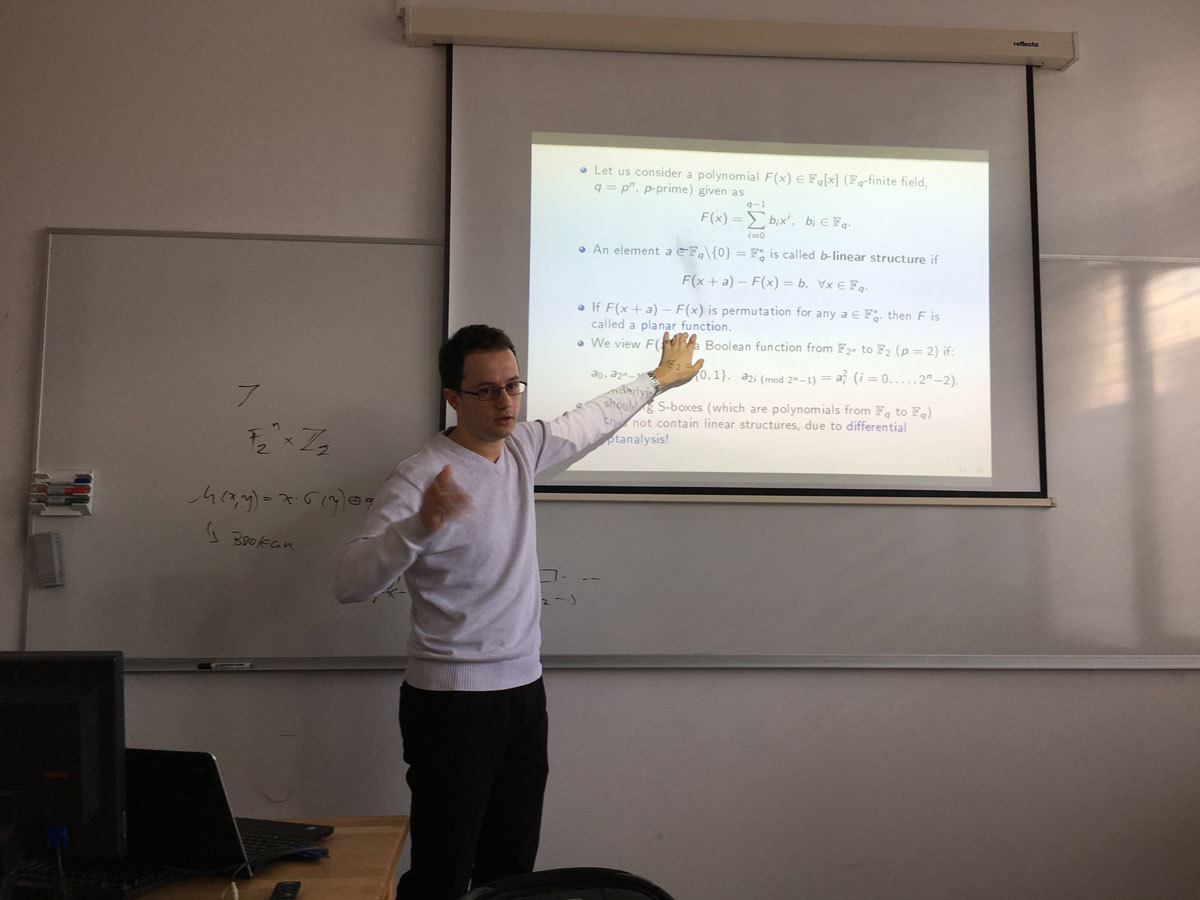Datum in ura / Date and time: 12.12.16
(10:00-11:00)
Predavalnica / Location: FAMNIT-POŠTA
Predavatelj / Lecturer: Rok Požar (UP FAMNIT, UP IAM)
Naslov / Title: The simultaneous conjugacy problem
Vsebina / Abstract:

Let S_n be the symmetric group on n letters. Given two r-tuples (a_1,a_2,...,a_r) and (b_1,b_2,...,b_r) of permutations of S_n the decision r-simultaneous conjugacy problem asks whether there is a permutation \tau in S_n which simultaneously conjugates these two tuples, that is, whether the following system of equations b_j = \tau^{-1}a_j\tau, j=1,2,...,r, over S_n has a solution. The search version of this problem is defined in the obvious manner, and is referred to as the search simultaneous conjugacy problem.

The solution of the simultaneous conjugacy problem has great potential for a variety of applications in different fields of mathematics and computer science such as automata theory, permutation groups, maps on surfaces, graph theory, representation theory, and cryptography. For example, in the language of deterministic finite automata the decision simultaneous conjugacy problem  is precisely the semiautomata isomorphism problem. The question whether two oriented maps on a closed surface are isomorphic translates to the special case of the decision simultaneous conjugacy problem for r = 2. Also, the decision problem interpreted in terms of permutation matrices is equivalent to a special case of asking whether two group representations are equivalent. An important variant of the conjugacy problem occurs when asking for all solution where a_j = b_j for all indices j; in this case we ask for the centralizer of a given set of permutations. In particular, this is encountered in finding the automorphism groups of embedded graphs on surfaces. Further, in the theory of covering graphs the question whether two covering projections are equivalent translates to the decision version of the conjugacy problem. Last but not least, the simultaneous conjugacy problem can be naturally generalised to arbitrary groups. For instance, in braid groups the search problem plays an important role in studying braid cryptographic protocols.

The best-known deterministic algorithm which solves the search version (and hence the decision version) has the \mathcal{O}(rn^2) time complexity. In addition, there are no lower bounds in the literature for the decision/search simultaneous conjugacy problem, except the trivial linear bound \mathcal{O}(rn). In the talk we present some new results which reduce the gap between the upper and lower bound.

Joint work with Andrej Brodnik and Aleksander Malnič.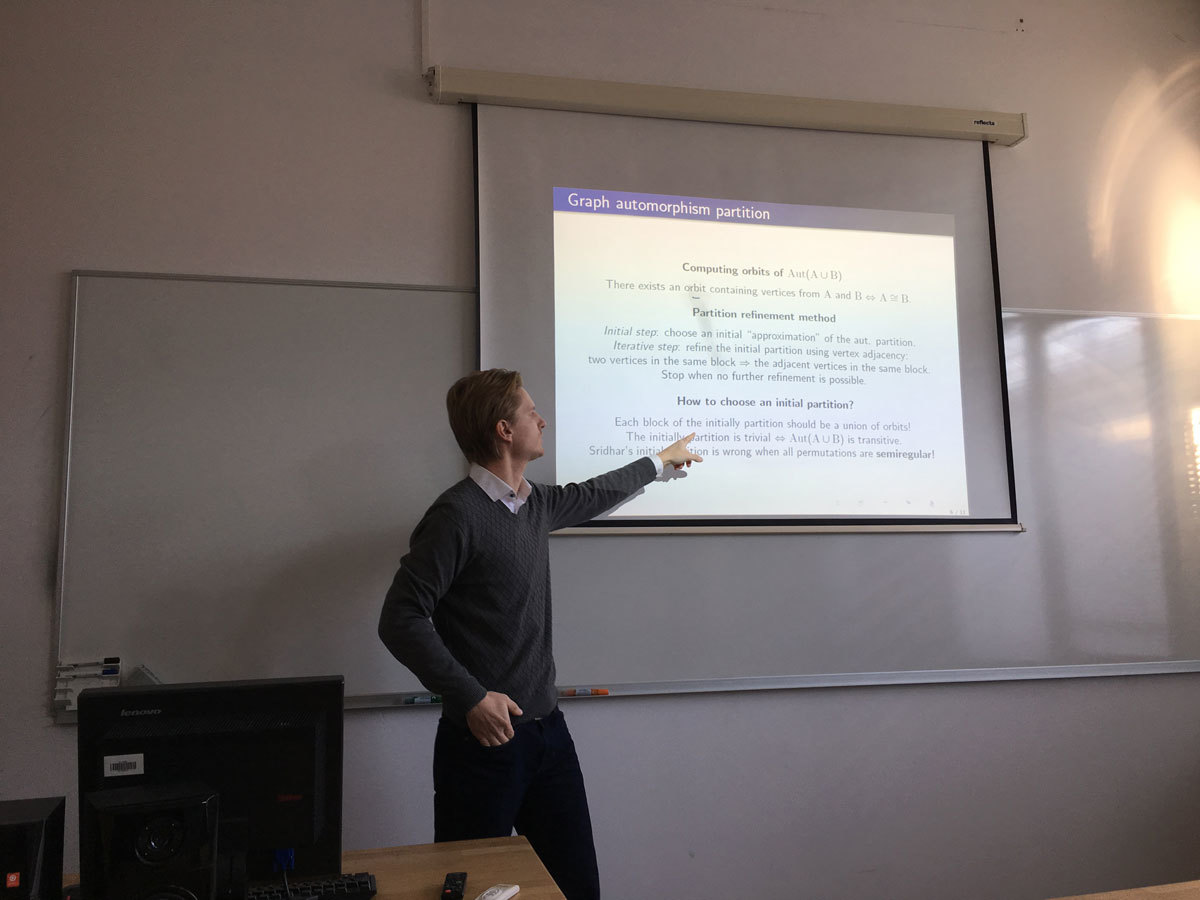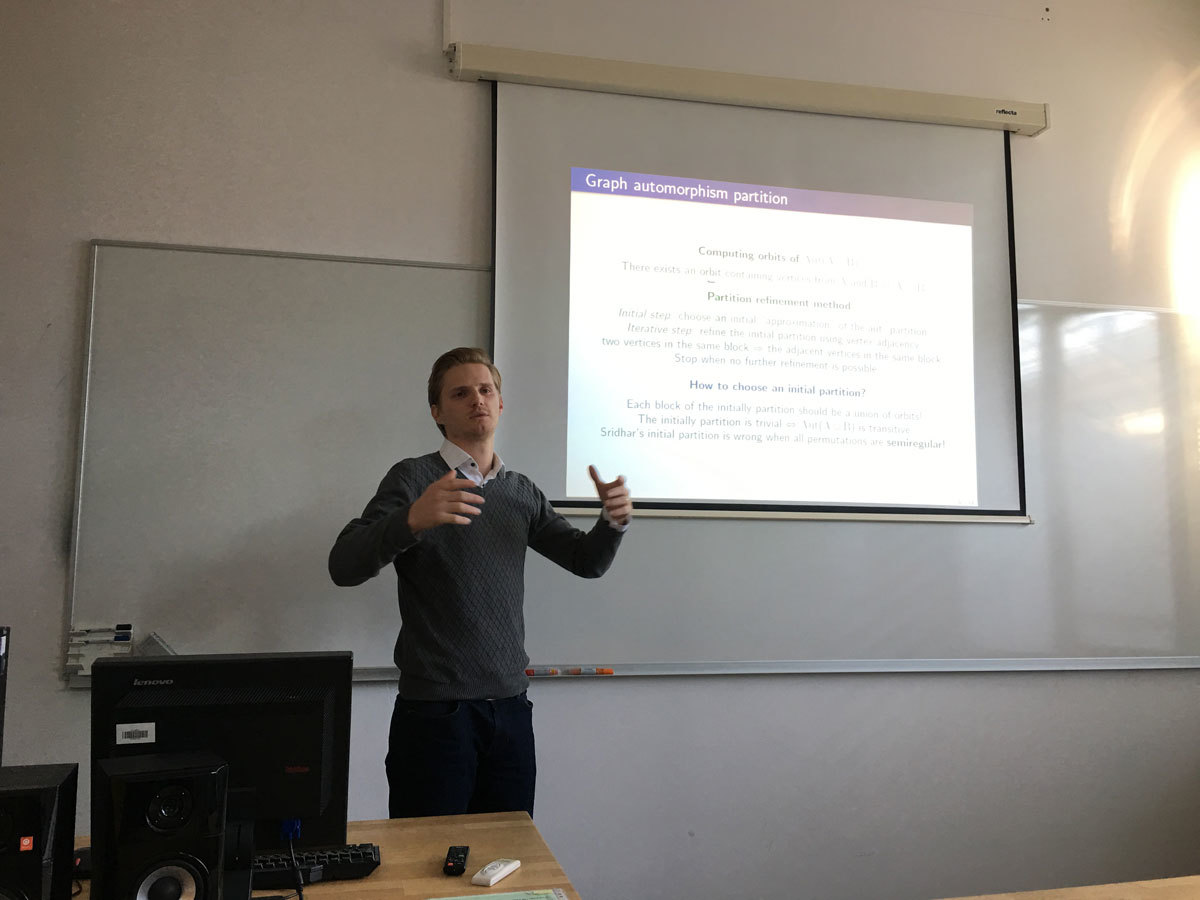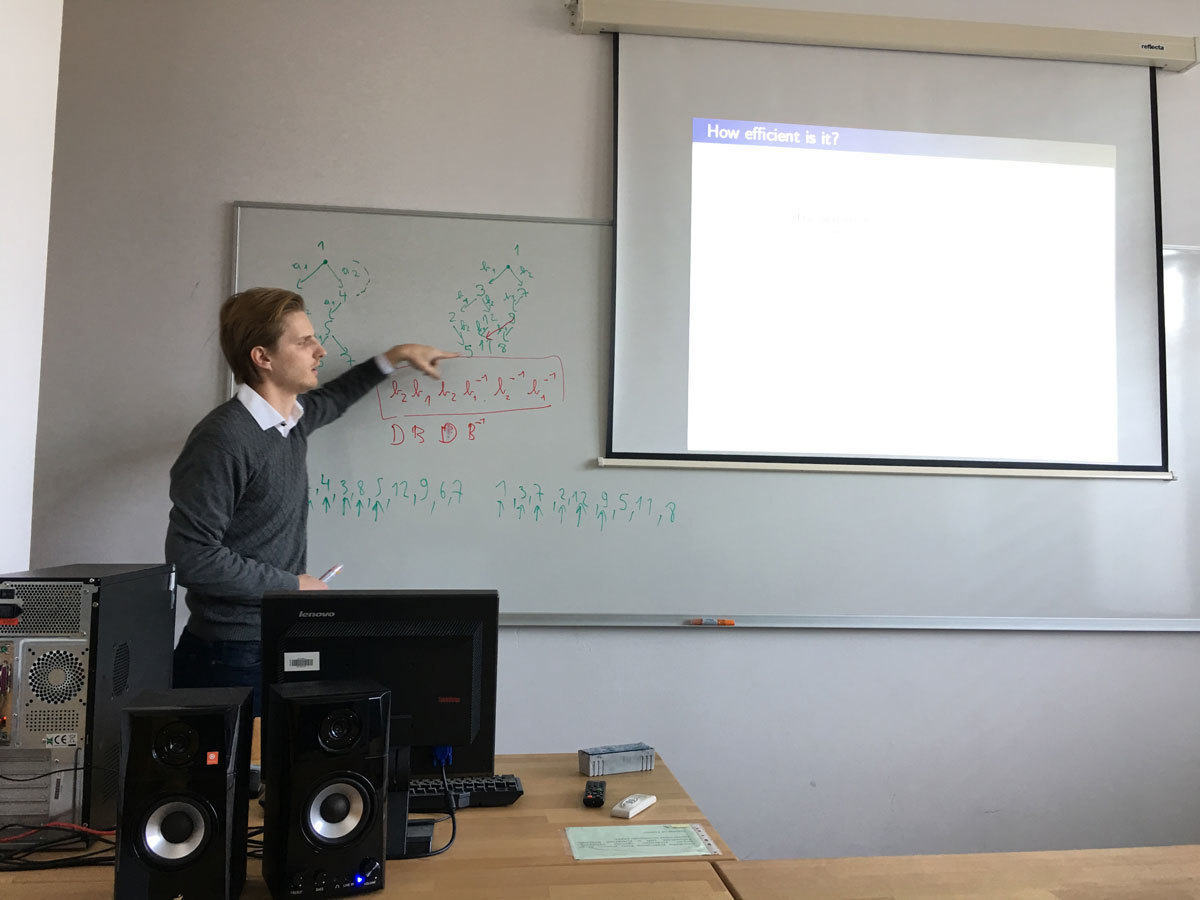Datum in ura / Date and time: 6.12.16
(9:30--10:30)
Predavalnica / Location: FAMNIT-Muzejski1
Predavatelj / Lecturer: Tınaz Ekim (Bogazici University, Istanbul, Turkey)
Naslov / Title: Characterization and Recognition of Stable Equimatchable Graphs
Vsebina / Abstract:

(Joint work with Zakir Deniz)

Our Turkish-Slovenian Project entitled “New Trends in Matching Theory” has started in July 2014 and will be finishing in January 1 st , 2017. We first summarize questions addressed in the framework of this project and the results obtained. We then focus on one of our most recent results about stable equimatchable graphs.

A graph G is equimatchable if every maximal matching of G has the same cardinality. We are interested in equimatchable graphs such that the removal of any edge from the graph preserves the equimatchability. We call an equimatchable graph G edge-stable if G-e is equimatchable for any e\in E(G). After noticing that edge-stable equimatchable graphs are either 2-connected factor-critical or bipartite, we characterize edge-stable equimatchable graphs. This characterization yields an O(min(n^{3.376} , n^{1.5} m)) time recognition algorithm. We also define vertex-stable equimatchable graphs and show that they admit a simpler characterization. Last, we introduce and shortly discuss the related notions of edge-critical and vertex-critical equimatchable graphs, pointing out the most interesting case in their characterization as an open question.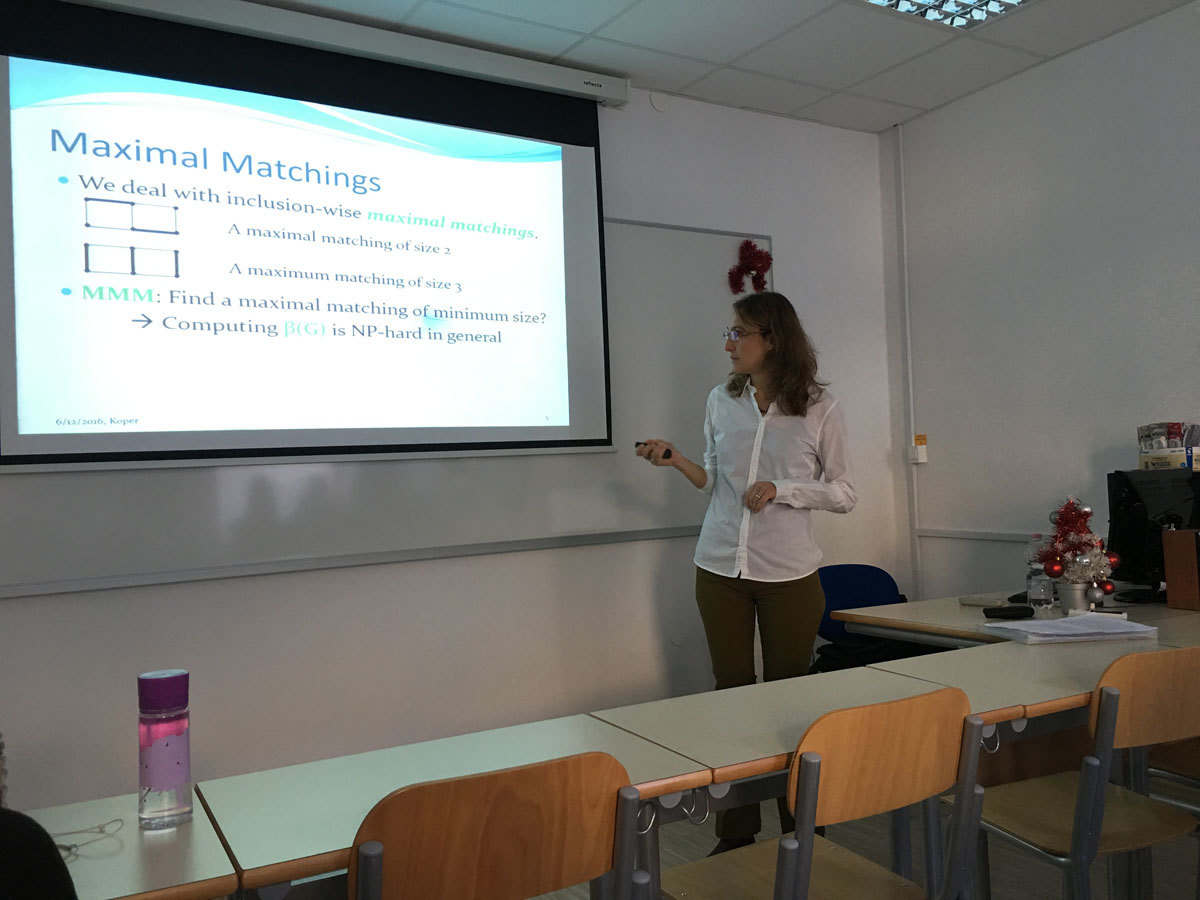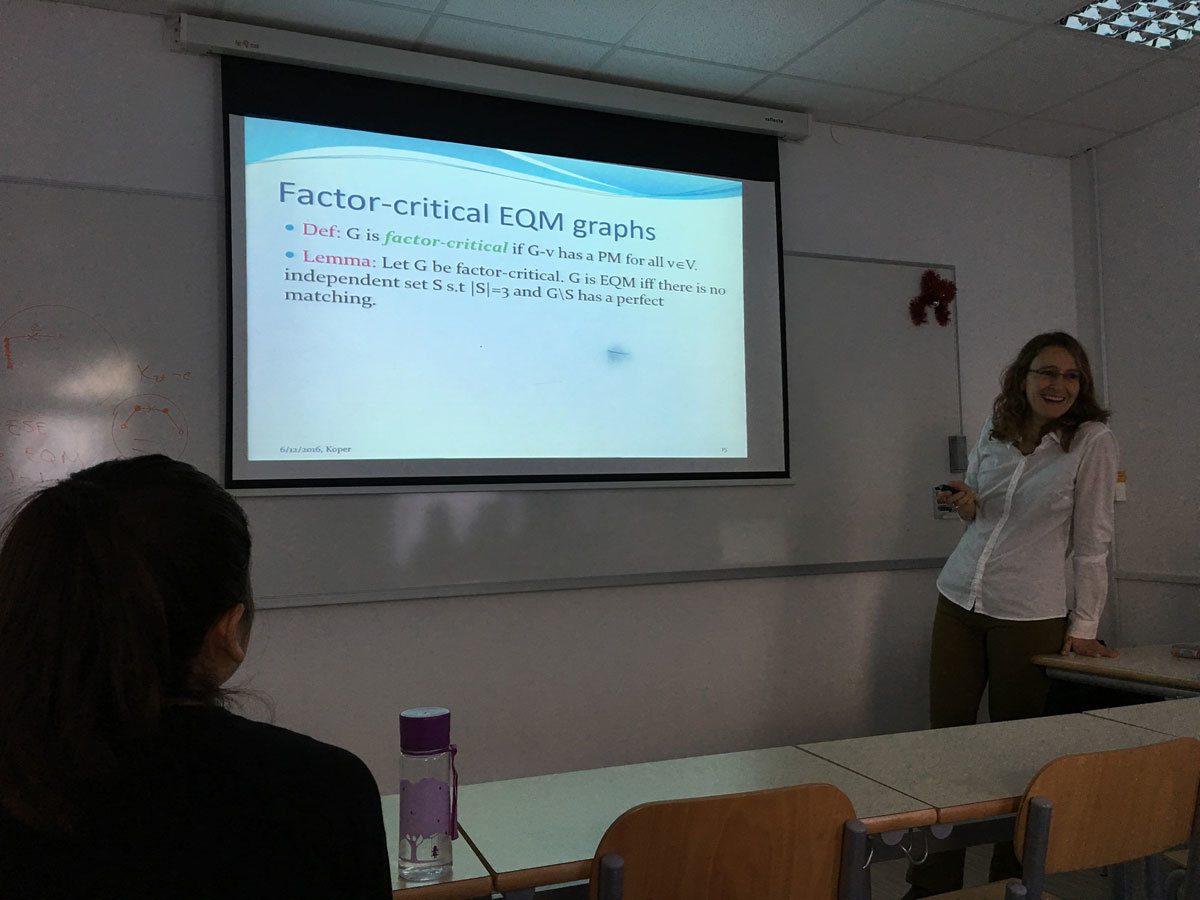Datum in ura / Date and time: 5.12.16
(10:00-11:00)
Predavalnica / Location: FAMNIT-POŠTA
Predavatelj / Lecturer: Sergey Goryainov (Chelyabinsk State University, Chelyabinsk, Russia; N.N. Krasovskii Institute of Mathematics and Mechanics UB RAS, Yekaterinburg, Russia.)
Naslov / Title: On eigenfunctions and maximal cliques of Paley graphs of order q^2
Vsebina / Abstract:

This is joint work with Vladislav Kabanov, Leonid Shalaginov and Alexandr Valyuzhenich.

Many combinatorial configurations (for example, perfect codes, latin squares and hypercubes, combi-
natorial designs and their q-ary generalizations — subspace designs) can be defined as an eigenfunction on a graph with some discrete restrictions. The study of these configurations often leads to the question about the minimum possible difference between two configurations from the same class (it is often related with bounds of the number of different configurations; for example, see [1–5]). Since the symmetric difference of these two configurations is also an eigenfunction, this question is directly related to the minimum cardinality of the support (the set of nonzero) of an eigenfunction with given eigenvalue. This talk is devoted to the problem of finding the minimum cardinality of the support of eigenfunctions in the Paley graphs of order q^2 (denote it by P(q^2)), where q is prime power. Currently, a similar problem is solved for Hamming graphs H(n, q) for q = 2 (see ). In  Vorob’ev and Krotov proved the lower bound on the cardinality of the support of an eigenfunction of the Hamming graph. In  the minimum cardinality of the support of eigenfunctions in the Hamming graphs with the second largest eigenvalue n(q − 1) − q was found.

In this talk, we present construction of eigenfuctions on P(q^2) for both non-principal eigenvalues; the cardinality of the support of the eigenfunctions is equal to q + 1. It follows from , that our construction gives eigenfunctions with minimum cardinality of support. As a related topic, we will discuss maximal cliques in P(q^2) of order (q + 1)/2 and (q + 3)/2, where q ≡ 1(4) and q ≡ 3(4), correspondingly. In , Baker, Ebert, Hemmeter and Woldar proposed contruction of such cliques, but they noted that their construction doesn’t cover all known cliques.

References

 E. F. Assmus, Jr and H. F. Mattson. On the number of inequivalent Steiner triple systems. J. Comb. Theory, 1(3):301-305, 1966.

 O. Heden and D. S. Krotov. On the structure of non-full-rank perfect q-ary codes. Adv. Math. Commun., 5(2):149-156, 2011.

 D. Krotov, I. Mogilnykh, V. Potapov. To the theory of q-ary Steiner and other-type trade. Discrete Mathe-
matics. 2016. V. 339, N 3. P. 1150-1157.

 V. N. Potapov. On perfect 2-colorings of the q-ary n-cube, Discrete Math., 2012, V. 312, N. 8, 1269-1272.  V. N. Potapov and D. S. Krotov. On the number of n-ary quasigroups of finite order. Discrete Math. Appl., 21(5-6):575-585, 2011.

 K. V. Vorob’ev and D. S. Krotov. Bounds for the size of a minimal 1-perfect bitrade in a Hamming graph. J. Appl. Ind. Math., 9(1):141-146, 2015. translated from Diskretn. Anal. Issled. Oper. 6(21):3-10, 2014.

 A. Valyuzhenich. Minimum supports of eigenfunctions of Hamming graphs. Accepted to Discrete Mathemat-
ics.

 R. D. Baker, G. L. Ebert, J. Hemmeter, A. J. Woldar. Maximal cliques in the Paley graph of square order. J. Statist. Plann. Inference 56 (1996) 33-38.